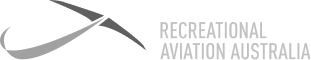Is the ratio of lift to weight and a measurement of stress or load that is added to an aircraft in flight. It seems as though the aircraft is adding weight, with an increase in load/stress. As an example, a load factor of 2 means that the apparent weight of the aircraft has doubled and this is due to a force applied on the aircraft.Is the ratio of lift to weight and a measurement of stress or load that is added to an aircraft in flight. It seems as though the aircraft is adding weight, with an increase in load/stress. As an example, a load factor of 2 means that the apparent weight of the aircraft has doubled and this is due to a force applied on the aircraft.

7 Day

FREE

Trial